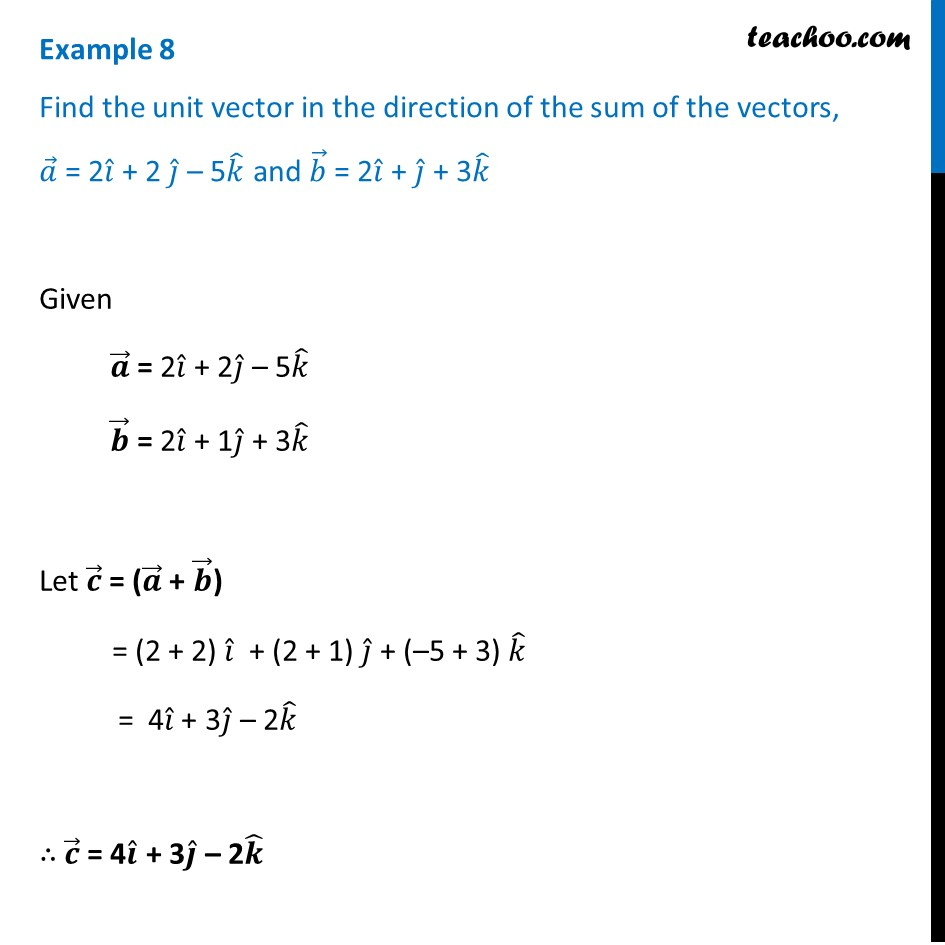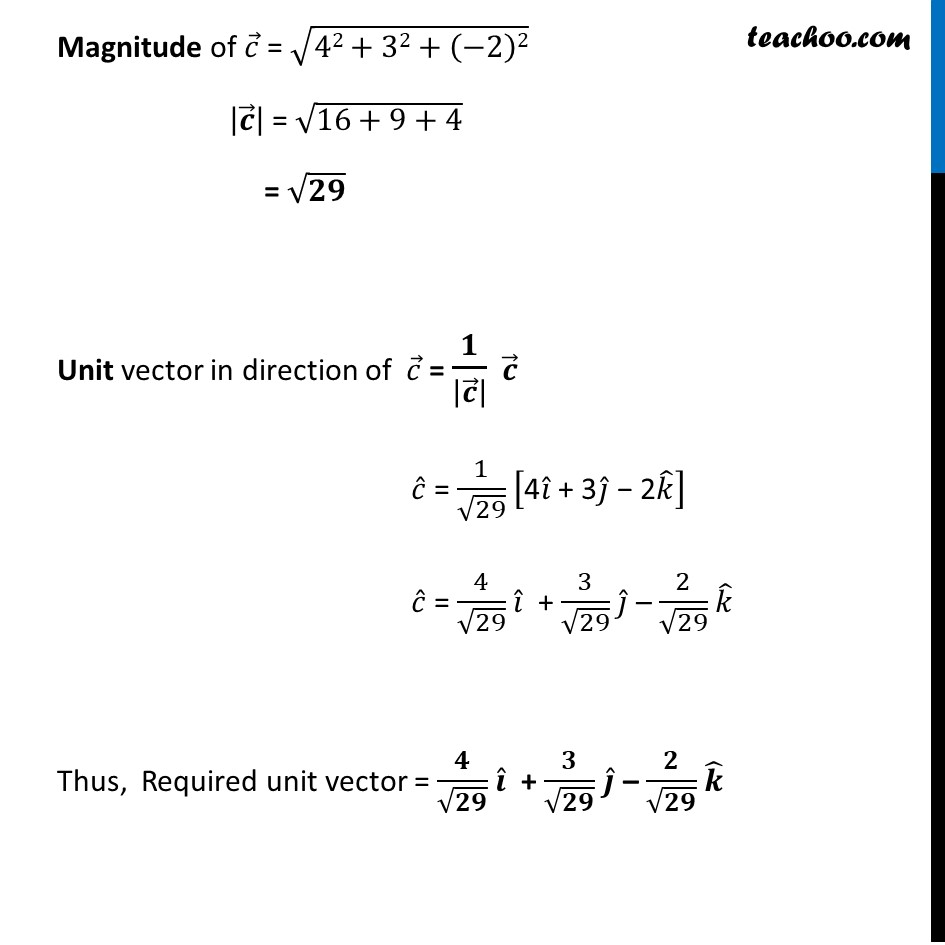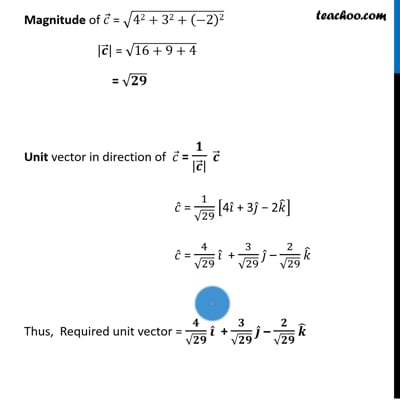Chapter 10 Class 12 Vector Algebra
Concept wiseThis video is only available for Teachoo black users

Introducing your new favourite teacher - Teachoo Black, at only ₹83 per month

### Transcript

Example 8 Find the unit vector in the direction of the sum of the vectors, 𝑎 ⃗ = 2𝑖 ̂ + 2 𝑗 ̂ – 5𝑘 ̂ and 𝑏 ⃗ = 2𝑖 ̂ + 𝑗 ̂ + 3𝑘 ̂ Given 𝒂 ⃗ = 2𝑖 ̂ + 2𝑗 ̂ – 5𝑘 ̂ 𝒃 ⃗ = 2𝑖 ̂ + 1𝑗 ̂ + 3𝑘 ̂ Let 𝒄 ⃗ = (𝒂 ⃗ + 𝒃 ⃗) = (2 + 2) 𝑖 ̂ + (2 + 1) 𝑗 ̂ + (–5 + 3) 𝑘 ̂ = 4𝑖 ̂ + 3𝑗 ̂ – 2𝑘 ̂ ∴ 𝒄 ⃗ = 4𝒊 ̂ + 3𝒋 ̂ – 2𝒌 ̂ Magnitude of 𝑐 ⃗ = √(42+32+(−2)2) |𝒄 ⃗ | = √(16+9+4) = √𝟐𝟗 Unit vector in direction of 𝑐 ⃗ = 𝟏/|𝒄 ⃗ | 𝒄 ⃗ 𝑐 ̂ = 1/√29 ["4" 𝑖 ̂" + 3" 𝑗 ̂" − 2" 𝑘 ̂ ] 𝑐 ̂ = 4/√29 𝑖 ̂" " + 3/√29 𝑗 ̂" "– 2/√29 𝑘 ̂" " Thus, Required unit vector = 𝟒/√𝟐𝟗 𝒊 ̂" " + 𝟑/√𝟐𝟗 𝒋 ̂" "– 𝟐/√𝟐𝟗 𝒌 ̂